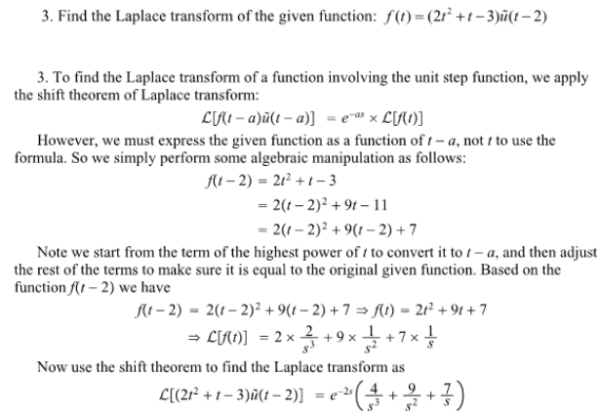Find the Laplace transform of the given function: f(t)=(2t^2+t-3)u(t-2).Find the Laplace transform of the given function: f(t)=(2t^2+t-3)u(t-2).

System Dynamics Page 2 dynamics dynamics dynamics dynamics dynamics dynamics dynamics System dynamics Page 3